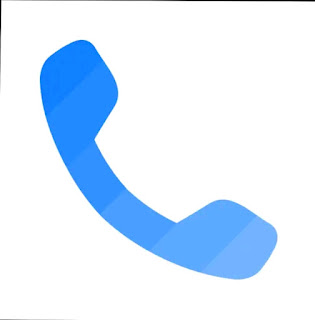## Wednesday, October 13, 2021

### Mind-Blowing: Amazing Puzzles with Answers Student Usful

Mind-Blowing: Amazing Puzzles with Answers Student Usful
A puzzle is a game, problem, or toy that tests a person's ingenuity or knowledge. In a puzzle, the solver is expected to put pieces together in a logical way, in order to arrive at the correct or fun solution of the puzzle.There are different genres of puzzles, such as crossword puzzles, word-search puzzles, number puzzles, relational puzzles, and logic puzzles.

Puzzles are often created to be a form of entertainment but they can also arise from serious mathematical or logical problems. In such cases, their solution may be a significant contribution to mathematical research.1 Horse sold out at Rs. 500
First Person :::: 4 Horses x Rs. 500
= Rs. 2000 -- Rs. 500
NEt Rs. 1500 For first Person

Second person ::: 2 horses x Rs. 500
== Rs. 1000
+ Rs. 500
Net Rs. 1500 For Second  person

Explanation:

In the given puzzle, the logic followed is

First digits comes from the number of letters in the word

Second digit is its serial number

Third digit is the addition of first and second digits

Etymology

The Oxford English Dictionary dates the word puzzle (as a verb) to the end of the 16th century. Its earliest use documented in the OED was in a book titled The Voyage of Robert Dudley...to the West Indies, 1594–95, narrated by Capt. Wyatt, by himself, and by Abram Kendall, master (published circa 1595). The word later came to be used as a noun, first as an abstract noun meaning 'the state or condition of being puzzled', and later developing the meaning of 'a perplexing problem'. The OED's earliest clear citation in the sense of 'a toy that tests the player's ingenuity' is from Sir Walter Scott's 1814 novel Waverley, referring to a toy known as a "reel in a bottle".

PUZZLE -

After performing the multiplication, we reach the result by adding the individual numbers that make up the result.

If 12x12 = 144, then 1 + 4 + 4 = 9

If 23x23 = 529, then 5 + 2 + 9 = 16

If 34x34 = 1156, then 1 + 1 + 5 + 6 = 13

The etymology of the verb puzzle is described by OED as "unknown"; unproven hypotheses regarding its origin include an Old English verb puslian meaning 'pick out', and a derivation of the verb pose.